# VBA Int / Integer Data Type (Dim Variable)

## Integer (Int) Variable Type

The VBA Int data type is used to store whole numbers (no decimal values). However as we’ll see below, the Integer values must fall within the range ‑32768 to 32768.

To declare an Int variable, you use the Dim Statement (short for Dimension):

``Dim intA as Integer``

Then, to assign a value to a variable, simply use the equal sign:

``intA = 30000``

Putting this in a procedure looks like this:

``````Sub IntExample()
'declare the integer
Dim intA as Integer
'populate the integer
intA = 30000
'show the message box
MsgBox intA
End Sub``````

If you run the code above, the following message box will be shown.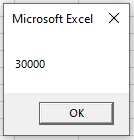### Long Variable Type

As mentioned above, Int variables can only store values between ‑32768 to 32768. If you attempt to assign a value outside that range to an Int variable you’ll receive an error: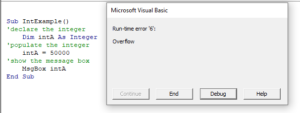When you click on de-bug, the code will break at the ‘populate the integer line as an integer cannot store a number as high as 50000.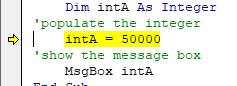Instead, you can declare a variable with the Long data type:

``Dim longA as Long``

Long Variables can store very long data types (-2,147,483,648 to 2,147,483,648).

#### Why would you use Int variables instead of Long variables?

Long variables use more memory. Years ago, memory was a big concern when writing code, however now computing technology is much improved and it’s doubtful you’ll encounter memory issues caused by long variables when writing VBA code.

We recommend always using Long variables instead of Int variables. We will continue this tutorial discussing Int variables, but keep in mind that you can use the Long variable type instead.

## Decimal Values & Int Data Types

Int variables can not store decimal values. If you pass a decimal number an integer, the decimal number will be rounded to remove the decimal.

Therefore, if you were to run the procedure below:

``````Sub IntExampleB()
'declare the integer
Dim intA as Integer
'populate the integer
intA = 3524.12
'show the message box
MsgBox intA
End Sub``````

You would get the following result (rounding down):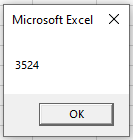However, this code below:

``````Sub IntExampleB()
'declare the integer
Dim intA as Integer
'populate the integer
intA = 3524.52
'show the message box
MsgBox intA
End Sub``````

Would return the following message box (rounding up):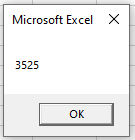### Decimal / Double Data Type

If you want to store a decimal place, you would need to declare a variable that allows for decimal places.  There are 3 data types that you can use – Single, Double or Currency.

``Dim sngPrice as Single``
``Dim dblPrice as Double``
``Dim curPrice as Currency``

The Single data type will round the decimal point slightly differently to the double and currency data type, so it is preferable to use double to single for accuracy.  A double can have up to 12 decimal places while currency and single can both have up to 4 decimal places.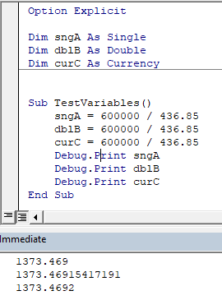For further information about these data types, you can have a look here.

## Declare Int Variable at Module or Global Level

In the previous examples, we’ve declared the Int variable within a procedure. Variables declared with a procedure can only be used within that procedure.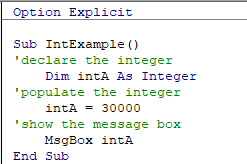Instead, you can declare Int variables at the module or global level.

### Module Level

Module level variables are declared at the top of code modules with the Dim statement.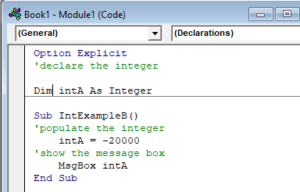These variables can be used with any procedure in that code module.

Stop searching for VBA code online. Learn more about AutoMacro - A VBA Code Builder that allows beginners to code procedures from scratch with minimal coding knowledge and with many time-saving features for all users!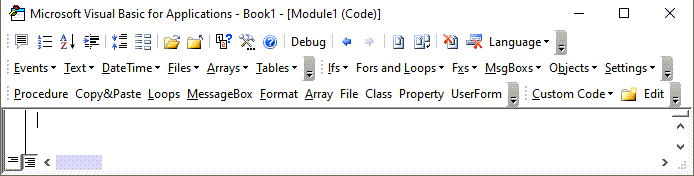### Global Level

Global level variables are also declare at the top of code modules. However, instead of using the Dim statement, use the Public statement to indicate that the integer variable is available to be used throughout your VBA Project.

``Public IntA as Integer``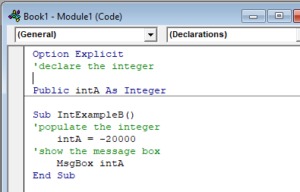If you were to declare the integer at a module level and then try to use it in a different module, an error would occur.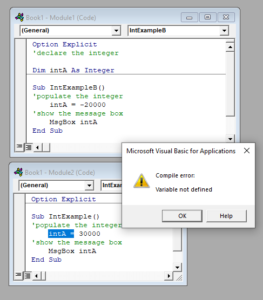However, if you had used the Public keyword to declare the integer, the error would not occur and the procedure would run perfectly.

## Convert String to Int

There might be an instance or instances when you will need to convert a number stored as a string to an integer value.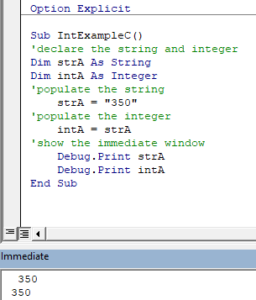You will notice in the immediate window that the integer value goes to the right indicating a number, while the string value goes to the left – indicating text.

## Convert Int to String

Conversely, you can convert an integer value to a string.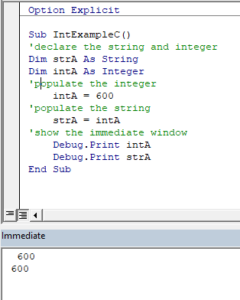For further information about these data types, you can have a look here.

VBA Programming | Code Generator does work for you!

### Format Integer Stored as String

To format Integers stored as string, use the Format Function.